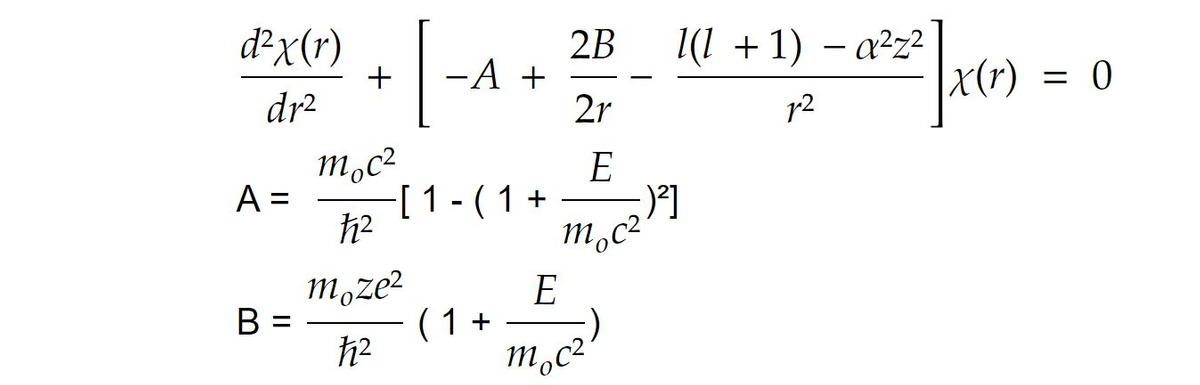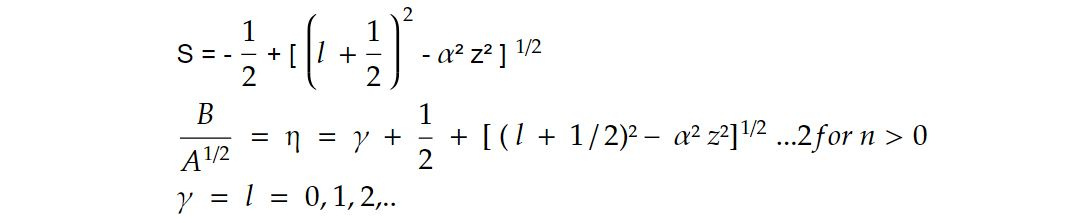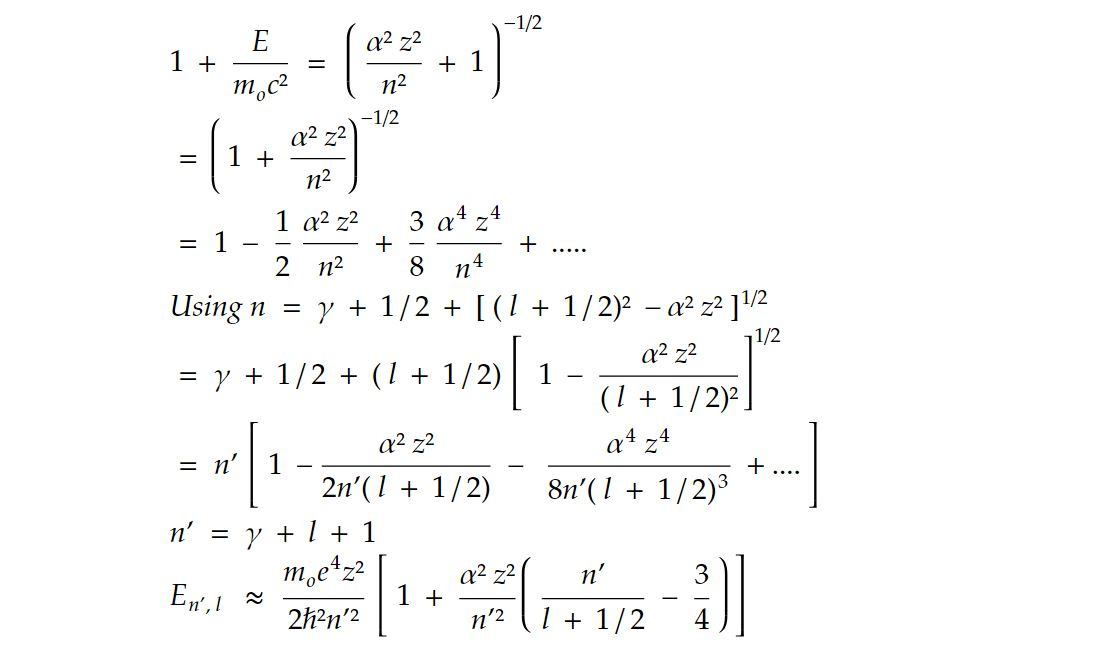# Bound states in a coulomb field

Bound states in a Coulomb field, also known as Coulomb bound states or hydrogen-like bound states, refer to the quantum mechanical solutions for particles (typically electrons) interacting with a central attractive Coulomb potential. The most famous example of this is the hydrogen atom, where an electron is bound to a nucleus (proton) due to their electrostatic attraction.

Here are some key points about bound states in a Coulomb field:

1. Coulomb Potential: The Coulomb potential describes the electrostatic interaction between charged particles and is given by:

V(r) = -k * e² / r

where V(r) is the potential energy, k is Coulomb's constant, e is the charge of the electron, and r is the distance between the electron and the nucleus.

2. Quantization: When dealing with quantum mechanics, the energy of a particle is quantized. The allowed energy levels for an electron in a Coulomb potential are described by the Schrödinger equation. Solving this equation results in a set of energy eigenstates, which represent the allowed bound states.

3. Energy Levels: The energy levels of a particle in a Coulomb potential are typically given by:

E_n = - (k² * e^4 * m)/(2 * ℏ²) * (1/n²)

where E_n is the energy of the nth bound state, m is the mass of the electron, ℏ is the reduced Planck constant, and n is a positive integer representing the principal quantum number.

4. Quantum Numbers: In addition to the principal quantum number (n), other quantum numbers like the azimuthal quantum number (l) and magnetic quantum number (m) are used to describe the various orbitals or states within an atom. These quantum numbers specify the shape and orientation of the electron's orbital.

5. Wave Functions: The solutions to the Schrödinger equation for the Coulomb potential are wave functions, typically expressed in terms of spherical harmonics and radial functions. These wave functions describe the probability distribution of finding the electron at various locations in space.

6. Hydrogen Atom: The hydrogen atom is the simplest example of a system with bound states in a Coulomb field. Its solutions are well-known and have been extensively studied. The quantum numbers n, l, and m determine the energy levels and the shapes of the electron orbitals.

Understanding the bound states in a Coulomb field is fundamental in quantum mechanics and is not limited to hydrogen atoms. It is a concept that applies to any system where a charged particle is bound by an attractive inverse-square law force, such as other atoms and ions with more than one electron. These bound states play a central role in understanding the behaviour of matter at the atomic and molecular scale.

Consider the motion of negatively charged spin zero particle in the coulomb field of a nucleus. It represents a π - meson atom. If the finite size of the nucleus is neglected.

e𝜙 = - ze²/ r

So𝜒 = rR and 𝛼² = e²/ cℏ for c² -> 𝛼

Again, we get the non relativistic result. Putting

𝜌 = zA^1/3 r

n = B/ A^1/2Then above equation can be written asS(S+1) = l (l + 1) - 𝛼²z² ....1

Putting n - S-1 = 𝛾 = 0,1,2...

n = 𝛾+S+1

Solve equation 1 for S and use the rootUsing these equation

B²/n² = AUnderstanding the bound states in a Coulomb field is fundamental in quantum mechanics and is not limited to hydrogen atoms. It is a concept that applies to any system where a charged particle is bound by an attractive inverse-square law force, such as other atoms and ions with more than one electron. These bound states play a central role in understanding the behaviour of matter at the atomic and molecular scale.

Bound states are crucial concepts in physics, particularly in the study of quantum mechanics and atomic and molecular physics. They represent a fundamental aspect of how particles and systems interact, and their importance can be understood in various contexts:

1. Stability of Matter: Bound states are essential for the stability of matter. In atomic and molecular physics, electrons are bound to the nucleus due to electromagnetic forces. Without these bound states, atoms and molecules would not exist, and the universe as we know it would be very different.

2. Energy Levels: Bound states are associated with discrete energy levels. In quantum mechanics, particles in bound states can only have certain quantized energy values. These energy levels are responsible for the discrete spectral lines observed in atomic and molecular spectra, which are essential for understanding the behaviour of matter and the composition of celestial objects.

3. Chemical Bonding: Bound states play a crucial role in chemical bonding. The formation of chemical bonds between atoms is essentially the result of electrons entering bound states that are shared between atoms. This bonding leads to the creation of molecules with distinct chemical properties, enabling the diversity of compounds and materials in the universe.

4. Nuclear Physics: In the nucleus of an atom, protons and neutrons are bound together by the strong nuclear force to form atomic nuclei. The stability and structure of atomic nuclei depend on the bound states of these nucleons. Understanding nuclear bound states is crucial for explaining nuclear reactions, nuclear stability, and the synthesis of elements in stars.

5. Particle Physics: In the realm of particle physics, bound states appear in the form of hadrons (composite particles made of quarks) and atomic nuclei (composite particles made of protons and neutrons). The study of bound states in particle physics is essential for understanding the fundamental forces and particles that make up the universe.

6. Astrophysics and Cosmology: Bound states have implications for the behaviour of matter in astrophysical and cosmological contexts. The formation of stars, planets, and galaxies relies on gravitational bound states, while the properties of dark matter and dark energy, which are thought to dominate the universe's energy content, also depend on the nature of bound states.

7. Technological Applications: Bound states are exploited in various technological applications. For example, in semiconductor devices like transistors, the behaviour of electrons in bound states is used to control electrical currents, enabling the operation of modern electronics.

In summary, bound states are fundamental to our understanding of the physical world, from the microscopic realm of quantum mechanics to the macroscopic scale of astrophysics and cosmology. They are central to explaining the stability, structure, and behaviour of matter, and their study has profound implications for science and technology.

This note is a part of the Physics Repository.